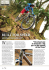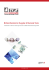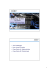# GREEDY SERVERS ON A TORUS

## Transcription

GREEDY SERVERS ON A TORUS
```Proceedings of the 2011 Winter Simulation Conference
S. Jain, R. R. Creasey, J. Himmelspach, K. P. White, and M. Fu, eds.
GREEDY SERVERS ON A TORUS
Karl W. Stacey
Dirk P. Kroese
School of Mathematics and Physics
The University of Queensland
Brisbane QLD 4072, Australia
[email protected]
School of Mathematics and Physics
The University of Queensland
Brisbane QLD 4072, Australia
[email protected]
Abstract
Queuing systems in which customers arrive at a continuum of locations, rather than at a finite number of
locations, have been found to provide good models for certain telecommunication and reliability systems as
well as dynamic stochastic vehicle routing problems. In this paper the continuum is the unit square, where
the opposite edges have been glued together to form a flat “torus”. Customers arrive according to a Poisson
process with arrival rate λ and are removed by servers. We investigate properties of the system under
various server strategies. We find that the greedy strategy, where a server simply heads for its closest point,
results in a stable system and we analyse the equilibrium distribution. The greedy strategy is inefficient,
in part because multiple greedy servers coalesce. We investigate the expected time until this occurs and
identify improvements to the greedy strategy.
1
Introduction
Much of the analysis of continuous server systems focuses on the situation where customers arrive on a
circle and are served by servers moving around the circle; see, for example, Fuhrmann and Cooper (1985),
Coffman and Gilbert (1986), Kroese and Schmidt (1992), Eliazar (2003), Altman and Foss (2004) and
Leskelä and Unger (2010). Some exceptions are Altman and Levy (1994), Bertsimas and van Ryzin (1991)
and Bertsimas and van Ryzin (1993), who investigate systems where customers arrive on a compact convex
n-dimensional space and are served by servers traveling through the space. For a review of continuous
queueing systems with greedy servers, see Rojas-Nandayapa, Foss, and Kroese (2011). Bertsimas and van
Ryzin propose a generic mathematical model for dynamic stochastic vehicle routing problems, in which the
aim is to minimise the average time that a customer spends in the system. They investigate and compare
a number of server strategies. This paper builds on this work, extending it in a number of directions.
Firstly, we use a toroidal topology (in 2 dimensions) rather than the Euclidean topology. This permits us to
simulate in an effectively boundaryless space, providing a natural extension of the circle (one dimensional
torus). Secondly, we consider extensions of the greedy strategy (referred to by Bertsimas and van Ryzin
as the nearest neighbour policy) both for single and multiple servers. Bertsimas and van Ryzin found
the greedy strategy to be the best of the strategies which they investigated. Thirdly, we provide empirical
relationships between the customer arrival rate and the time until multiple greedy servers converge as well
as the customer arrival rate and the long-run mean number of customers on the torus. In addition to the
Stacey and Kroese
application areas mentioned above, the model can be applied to biological systems where the (potentially
large number of) customers could represent, for example, individual plants in an outbreak of an introduced
species of vegetation. The boundarylessness of the torus might be appropriate in such a situation.
For the case of a server on the circle, if the server moves continuously in one direction around the circle,
removing customers instantly upon reaching them, the time that an arbitrary customer exists before being
removed is bounded by 2πr
v , where r is the radius of the circle and v is the speed of the server. In a space
with dimension greater than one, there is no analogous methodical manner in which a server can move
through the space and in doing so, reach each customer in the space in bounded time. Servers will need to
actively travel towards customers. The absence of a methodical manner, in which a server can sweep up
all customers in bounded time, suggests the following questions. Does there exist a server strategy such
that the system is stable? If so, how does stability depend on the customer arrival rate λ? And what is the
minimum average number of customers in the system that can be achieved by an optimal strategy? Altman
and Levy (1994) consider fairness criteria such as First-Come-First-Served (and variants of this, such as
First-Come-First-Served restricted to customers within a certain radius of the server’s current position). In
this paper, the goal of the servers is simply to minimise the mean time that an arbitrary customer spends on
the torus. For a stable system, this is equivalent to minimising the long-run expected number of customers
on the torus. Another interesting question relating to a stable system is: What is the equilibrium distribution
of the number of customers in the system and how does this depend on the arrival rate λ?
We find empirically that the greedy strategy results in a stable system for all arrival rates λ, where
the number of customers in equilibrium is right-skewed with a mean that is quadratic in λ. We find
empirically that the mean time until two greedy servers coalesce, after initially being maximally separated,
is asyptotically linear in λ.
The rest of the paper is organized as follows. Section 2 describes the model, offers some rules of thumb
for effective server strategies, and provides a method for simulating the system. In Section 3 we introduce
a method to simulate the greedy strategy. We then discuss the time until greedy servers coalesce, the
stability of the system under a greedy strategy, and the equilibrium distribution of the number of customers
on the torus. Some improved server strategies are discussed in Section 4. Section 5 provides a numerical
comparison of these strategies.
2
The Model
Let E = [0, 1]2 be the unit square and let T be a torus generated from E by identifying the point (0, y) as
being the same as the point (1, y) for all y ∈ [0, 1] and the point (x, 0) as being the same as the point (x, 1)
for all x ∈ [0, 1]. Customers, henceforth referred to as points, arrive according to a Poisson process with
rate λ per unit of time. Once a point arrives, it is assigned a random position on the torus. The positions
of points are independent and have a uniform distribution on T. On T live n servers. At any point in
time, each of the servers is doing one of two things, either (i) waiting at its current position or (ii) moving
at a constant speed in a straight line. In particular, the paths of the servers are piecewise linear. When a
server reaches the position of a point, the point is removed instantly. Without loss of generality, we let the
speed of the servers be 1 and consider what happens when λ is varied. The zero service time justifies this
approach, since with instantaneous service it is the ratio of the point arrival rate (λ) to server speed which
is the relevant variable. The actual movement of the servers depends on their strategy. A possible strategy
is the greedy strategy, where each server moves towards the nearest point in a straight line. This can be
reasoned to be suboptimal. If two servers travel towards the same point, one will reach the point first and
the other will have wasted travel time, during which it could have been moving towards another point.
The concept of servers being “closest” to points requires that a metric on the torus is specified. On a
torus, if the server moves off the top, he appears at the bottom and if he moves off the left, he appears at
the right and vice versa. We define a metric by
dT (x, y) = min{kx − yk , kx − y ± (1, 0)k , kx − y ± (0, 1)k , kx − y ± (1, 1)k , kx − y ± (−1, 1)k},
where k·k is the Euclidean norm, and x = (x1 , x2 ), y = (y1 , y2 ) ∈ T.
Stacey and Kroese
Figure 1 illustrates the concept. What we do is tile the torus’ square to make a large square of 3 × 3
torus squares. We now have nine x points and nine y points. We consider the Euclidean distances from the
x point in the centre square to all nine y points and take the minimum of these distances as our distance
between x and y. From now on, any reference to a distance or a metric will refer to this metric on the
torus.
Figure 1: Tiling to find the shortest distance between two points on a torus. The stars correspond to a single
server and the dots correspond to a single point, to which the server is heading. The solid line indicates
the shortest route.
The calculation of the torus metric can be simplified by noting that the square view of the torus is
offering a snapshot, via a viewing window, of an underlying lattice in R2 . Regardless of how we translate
this viewing window, each point will be visible exactly once. If we translate the viewing window such
that the server is at the position (0.5, 0.5), then the shortest path from the server to any point lies entirely
within the viewing window. Now rather than computing nine distances and finding the shortest, we only
need to compute one.
Figure 2: Simplifying the torus metric calculation. The viewing window is moved from the original square
with the solid border to the square with the dotted border. Note the shortest path from the server to the
point lies entirely within the square with the dotted border.
The positions of the points at time t are described by a counting measure Ct where Ct (A) indicates the
total number of points (at time t) in the set A ∈ B(T) and B(T) comprises the borel sets generated by the
torus metric on T. The position of the servers at time t is described by a vector ~St = (St,1 , ..., St,n ). Thus
the continuous server system can be described by the random object (Ct , ~St , t > 0) which takes values in
C × Tn , where C is the set of all counting measures on T.
The servers’ goal is to minimise the mean time that an arbitrary customer spends on the torus. For
d
a stable system, the total number of points on the torus converges in distribution; that is Ct (T) → C for
some random variable C, interpreted as the steady-state number of waiting points. The servers’ goal is to
Stacey and Kroese
minimise E C. Note that it is not obvious that the system is stable, although we find experimentally that
it is.
In attempting to minimise E C, servers can follow a large variety of strategies, some of which require
a large amount of information on the positions of the points and the movements of the other servers. We
assume the servers are omniscient; that is, they have complete knowledge of the system. The strategies
that we consider in this paper require, however, only partial knowledge of the system.
Even with a single server, the optimal strategy is not clear. Does the problem reduce to solving a
traveling salesman problem every time a new point arrives, or should the server follow a “self-avoiding”
path, where its movement is biased away from regions where it has already been towards regions where
the point density is greatest? Intuitively, taking account of differences in the point density over the torus
becomes less important, the fewer the number of points on the torus.
With multiple servers, the optimal strategy is still less clear, since each server’s actions affect the best
actions of the other servers. A good starting point is to specify that the server obeys some sensible rule
such as the following.
1. No server should move towards a point that another server will reach first.
2. Servers should collectively serve points in a “sensible” order. In particular, if there are m points
currently on the torus andPri is the remaining time until the ith point is removed, the servers should
move so as to minimise m
i=1 ri . This is a variant on the traveling saleman problem. It will mean
moving to denser areas first, so as to serve more points per unit time.
3. Since new points arrive uniformly distributed on the torus, the servers should ideally be maximally
spread out such that the expected distance of a new point from the closest server is minimised.
The third rule of thumb is not always compatible with the second; that is, to minimise the total of the
waiting points’ service times. For example, if there is a cluster of points in one area, the time the closest
server takes to reach and serve them all may be more than the time it takes for far away servers to reach
the cluster and start serving some of them. If the far away servers help, the points will be removed faster
but then the servers will be bunched up and the expected distance of a new point from the closest server
will not be minimised. Hence future points will take longer to remove. The optimal strategy will involve
a balance between serving the current points as quickly as possible and keeping the servers well spread
out. Note that this is only an issue when there are multiple servers. For a single server, any point on the
torus is as good as any other when minimising the expected distance to a point that is yet to arrive.
For a single server, an important point to make is that the actual position of the server on the torus is
irrelevant to the analysis. We can always translate the viewing window such that the server is at (0.5, 0.5).
It is the position of the points relative to the server that is important. Such a viewpoint has proved to be
very beneficial for the analysis of continuous server systems on a circle, c.f. Kroese and Schmidt (1992).
For multiple servers, the positions of the servers relative to each other are important. Multiple servers
will want to spread themselves out, such that the expected distance of a new point from the closest server
is minimised. For the single server, any server location is as good as any other in terms of minimising the
expected distance to a new point.
To simulate the servers in action, we need to keep track of point arrivals as well as servers arriving at
points. The following algorithm can be used for any server strategy by changing the allocation algorithm
at Steps 1, 4 and 5.
Algorithm 2.1 (Server Simulation)
Let si represent the position of the ith server and let pj represent
the position of the jth point. Start with a single point. Generate its position p1 uniformly on T. Let the
total number of points to have arrived be N = 1 and the total time elapsed be T = 0. Let ai be the index
of the point to which server i is allocated. Let δi be the distance of server i from its allocated point, pai ,
and let di be the direction that server i must travel in to reach pai . Let τi be the time remaining until
event i occurs, let ℓi be the location of this event and let ei be the type of the event, either ‘server arrival
at a point’ or ‘server arrival at space’ or ‘point arrival’.
Stacey and Kroese
1. Allocate the servers according to some allocation strategy.
2. Construct a list of upcoming events. This contains one event for each server arriving at its next
point (events 1 to n) and one event for the next arrival of a new point (event n + 1). For each
event i = 1, ..., n + 1, store: the time until it occurs, τi = δi for i = 1, ..., n and τn+1 ∼ Exp(λ);
the location, ℓi = si + τi di mod1, i = 1, ..., n and ℓn+1 ∼ U[0, 1)2 ; and the type of event, ei .
3. Find the next event by sorting the list of upcoming events to find the event where
τi = mini=1,...,n+1 τi . Let k be the index of that event and set T = τk . If the next event type, ek ,
is a server arrival, go to Step 4; otherwise, if the next event type is a point arrival, go to Step 5.
Set T = T + τk .
4. (SERVER ARRIVAL EVENT) Remove the point at pk . Update the server positions by setting
si = si + T di mod1, i = 1, ..., n. Reallocate all servers according to the allocation strategy.
Record di , ai and δi for i = 1, ..., n. Update the list of upcoming events as follows. Set τi = di
for i = 1, ..., n and τn+1 = τn+1 − T . Set the locations, ℓi = si + T di mod1, i = 1, ..., n. If
necessary (not necessary for the greedy strategy), reset the type of event, i.e. ei =‘server arrival
at a point’ or ‘server arrival at space’. Go to Step 3.
5. (POINT ARRIVAL EVENT) Update the server positions by setting si = si + T di mod1, i =
1, ..., n. Generate a U[0, 1)2 random vector and append it to the list of points. Set N = N + 1.
Reallocate all servers according to the allocation strategy. Record di , ai and δi for i = 1, ..., n.
Update the list of upcoming events as follows. Set τi = δi for i = 1, ..., n and τn+1 ∼ Exp(λ).
Set the locations, ℓi = si + T di mod1, i = 1, ..., n. If necessary (not necessary for the greedy
strategy), reset the type of event, i.e. ei =‘server arrival at a point’ or ‘server arrival at space’.
Go to Step 3.
We stop when we reach some maximum number of points or some time limit. Note that under some
strategies, such as the greedy strategy, it is only necessary to reallocate those servers who were heading
for the point that was just reached in Step 4. The other servers’ directions will remain unchanged until
they reach their point or a new point arrives.
3
The Greedy Strategy
Recall that under the greedy strategy each server is assigned to the point closest to it. When there are
no points on the torus, the servers stay in their current positions. The allocation strategy for the greedy
servers can be summarised as follows.
Algorithm 3.1 (Allocation Strategy: Greedy Server)
Let si , pj , δi , di , and ai be as in Algorithm 2.1. Let p be the number of points on the torus. Define an an “offset” vector Oi = (0.5, 0.5) − si .
1. If there are no points on the torus (p = 0), set the servers’ directions di to (0, 0) for all i = 1, ..., n
and stop; otherwise, proceed with the following steps.
2. For each server i = 1, ..., n, create a new set of points,
{pi,j , j = 1, ..., p} = {pj + Oi mod1, j = 1, ..., p}.
3. For j = 1, ..., p, let δi,j = kpi,j − (0.5, 0.5)k be the distance from server i to point j.
4. Set δi = minj=1,...,p δi,j be the distance of server i from its closest point. Let ai be the corresponding
index of this point.
5. To each server i, assign a direction di = (pi,ai − (0.5, 0.5))/δi .
Servers following the greedy strategy do not follow any of the sensible rules of thumb specified in
Section 2. The first rule (no server should move towards a point that another server will reach first) is
clearly violated. Servers also do not serve points in a sensible order (the second rule of thumb). However,
the most important drawback of the greedy strategy is that the servers coalesce (end up on top of each other)
very quickly and, therefore, might as well be considered as a single server from the time of coalescence
Stacey and Kroese
onwards. Consider the series of plots in Figure 3, where the servers are represented as pacmen chasing
the points which are represented as cherries. The thick dashed lines represent the directions in which the
pacmen are moving and the dotted lines represent the paths along which they have moved so far.
Figure 3: Coalescence of greedy servers.
3.1 Server Coalescence Times Under the Greedy Strategy
Our empirical observations are that for a higher point arrival rate λ the coalescence of the greedy servers
is slower. Intuitively this makes sense, since in order to coalesce, servers will need to be heading towards
the same point and/or different points that are quite close together but at some stage they must be heading
toward the same point. The more points on the torus, the less likely that any two servers at different given
positions will be heading towards the same point. The coalescence of two greedy servers was investigated
via Monte Carlo simulation. For the purposes of the simulation, coalescence was defined to have occurred
when the two servers reached within 10−5 of each other. We find that the number of point arrivals before
coalescence appears to be asymptotically quadratic in the point arrival rate λ and that the time before
coalescence appears to be asymptotically linear in the point arrival rate λ. Figure 4 shows the mean
number of point arivals until coalescence for various λ values. A quadratic trendline is also displayed. The
corresponding R2 for the fit was 0.9997. The data for Figure 4 was generated from 10000 simulation runs
until coalescence using λ = 1, ..., 15. The two servers were initially maximally separated.
Mean number of arrivals Nc before
the two servers are within 10−5
70
60
data
Nc = 0.15813λ2 + 1.0644λ + 13.941
50
40
30
20
10
0
5
10
15
λ
Figure 4: Mean number of arrivals before coalescence of two greedy servers, starting with one point as
per Algorithm 2.1.
Stacey and Kroese
Figure 5 shows the mean time until coalescence for various λ values. A linear trendline was fitted,
excluding the first 20 observations. The corresponding coefficient of determination for the fit was 0.9159.
The data for Figure 5 was generated from 1000 simulation runs until coalescence using λ = 1, ..., 300.
The two servers were initially maximally separated.
50
Time tc until coalescence
λ = 1, ..., 20
λ = 21, ..., 300
40
linear trend
30
tc = 0.12578x + 2.4013
20
10
0
0
50
100
150
200
250
300
λ
Figure 5: Mean time until coalescence of the two greedy servers, starting with one point as per Algorithm 2.1.
The aberrant behaviour in the earlier observations of Figure 5 could be due to the servers waiting a
significant time with no points on the torus. This effect disappears as the point arrival rate increases.
3.2 When Does the Greedy Strategy Lead to a Stable System?
Equilibrium mean number of points Ne
Since it seems that for any finite λ, the greedy servers do eventually coalesce to the same positions, a
stable system with n servers will in the long-run be equivalent to a stable system with a single server.
Hence, without loss of generality, we can restrict ourselves to the case of a single greedy server. We find
empirically that at equilibrium the mean number of points on the torus is a quadratic in λ (see Figure 6).
16000
14000
12000
data
Ne = 0.385λ2 − 2.63λ
10000
8000
6000
4000
2000
0
40
60
80
100
120
140
160
180
200
λ
Figure 6: Equilibrium mean number of points for λ = 40, 80, 120, 160, 200. A quadratic trend line through
the origin is fitted.
Stacey and Kroese
Mean number of points at the N th arrival
This makes intuitive sense. The number of points on the torus will increase until such time that the mean
distance between each point and the closest next point is equal to the reciprocal of the arrival rate λ. Since the
torus is a two-dimensional space, multiplying λ by a factor k causes the required number of points to change
by a factor k 2 . Figure 6 displays the equilibrium mean number of points for λ = 40, 80, 120, 160, 200. We
also know the mean will be 0 for λ = 0. Hence, a quadratic trend line through the origin is fitted and the
R2 for the fit is 0.999993. For each λ, the system was simulated in equilibrium for 5 × 106 point arrivals.
The total time spent in each state (number of points) was recorded and the equilibrium mean number of
points was estimated from this information.
To gain an idea of the evolution of the mean number of points in the system, starting from a single
point until the system reaches equilibrium, Figure 7 displays, for various λ, the mean number of points on
the torus at the time of the N th arrival (the middle lines). The two lines on either side of the middle line
are upper and lower 95% confidence bounds. The data for Figure 7 was generated from 1000 simulation
runs of 1000 point arrivals in each run, for λ = 1, 2, ..., 10. Equilibrium was reached by 1000 point arrivals
for each of these λ values.
40
30
20
10
0
10
8
1000
6
800
600
4
400
2
λ
0
200
0
N
Figure 7: Mean number of points on the torus at the time of the N th arrival for λ = 1, 2, ..., 10.
What the plot of the mean number of points on the torus does not tell us is how the number of points
on the torus fluctuates in equilibrium.
3.3 What is the Equilibrium Distribution of the Number of Points waiting for service?
Figure 8 depicts the results of a simulation run with a single greedy server and an arrival rate of λ = 200.
The number of points waiting for service on the torus appeared to reach an equilibrium of around 14900
points after 100,000 point arrivals. It was run for a total burn-in period of 1 million point arrivals and from
that point onwards the total time spent at each number of points on the torus was recorded for a further
50 million arrivals. The empirical probability mass function that was generated from the data is shown
normalised in Figure 8. A kernel density estimate is fitted.
Stacey and Kroese
0.45
0.4
0.35
0.3
0.25
0.2
0.15
0.1
0.05
0
−3
−2
−1
0
1
2
3
4
5
Figure 8: A normalised version of the empirical probability mass function of the steady state number of
waiting points on the torus C for a single greedy server and an arrival rate λ = 200.
Figure 9 provides an indication of the spatial distribution of points in equilibrium for an arrival rate of
200.
Figure 9: A snapshot in equilibrium of the positions of waiting points on the torus for a single greedy server
and an arrival rate λ = 200. The frame of reference has been shifted such that the server is at (0.5,0.5).
Stacey and Kroese
An interesting observation from Figure 8 is that the distribution is positively skewed. Perhaps this is
due to the fact that, at low point levels when the drift is upwards, the arrival rate is constant at λ, regardless
of the server’s position on the torus, whereas at high point levels when the drift is downwards, the rate
at which the server removes points is highly dependent on its position. If the server happens to be in
a relatively sparse area of the torus when the point levels are high, the high levels will persist until the
server reaches a denser region. Under the greedy strategy, the server will eventually be drawn to the denser
regions but new points that land close to him and on the wrong sides can disrupt his progress.
4
Better Strategies
The greedy strategy has several important drawbacks related to its violation of sensible rules of thumb for
server behaviour. The coalescence of greedy servers, in particular, is a significant problem but one that
can be easily solved, for example, by the strategy we term “simple sharing”.
4.1 Simple sharing
Simple sharing is a simple improvement on the greedy strategy which prevents servers from coalescing.
The allocation algorithm is as follows.
Algorithm 4.1 (Allocation Strategy: Simple Sharing)
1. Allocate each server individually to a point via the greedy strategy. If there are no points on the
torus, the servers stay where they are.
2. Look for clashes where two or more servers are allocated to the same point. If there are none,
stop (the servers have been allocated); otherwise, go to Step 3.
3. For the servers which are not the closest to the point they have been allocated, add the point to
which they would be heading to a ’taboo list of points’ for that server. Reallocate these servers
via the greedy strategy, over the set of points excluding those on their taboo lists. If, for a server,
there are no points on the torus which are not on the taboo list, the servers stay where they are.
Go back to Step 2.
It does not make much sense to compare the performance of the simple sharing strategy to the
performance of the greedy strategy since under the greedy strategy, multiple servers very quickly coalesce
to be one. Therefore, any comparison with multiple servers would seem unfair, and with a single server
the two strategies are equivalent. Perhaps it is best to accept the simple sharing strategy as strictly better
than the greedy one and to use this as a base to compare with more advanced strategies.
In the simple sharing strategy, the servers only look one step ahead. Say there are two servers and two
points. Server 1 is closest to point 1, but server 1 could also reach point 2, going via point 1, faster than
server 2 could reach point 2. The simple sharing strategy would allocate server 1 to point 1 and server 2 to
point 2. The servers need to look 2 steps ahead here. “m-step ahead sharing” extends the simple sharing
strategy in this way. The algorithm can be programmed as follows.
Stacey and Kroese
Algorithm 4.2 (Allocation Strategy: m-Step Ahead Sharing)
1. If there are no points on the torus, the servers stay where they are. Stop the algorithm; otherwise,
for each server i, create a list Fi,1 = {1, 2, ..., p} of points that they can visit on the first step
(Initially this includes all points). Allocate each server individually via the greedy strategy over
the set of points Fi,1 . Let ai,1 be the index of the point, to which server i is allocated. For each
server i, create a list Fi,2 = {1, 2, ..., p} \ {ai,1 } of points that server i can visit subsequently.
Then for j = 2, ..., m,
(a)
If Fi,j is empty, stop and go to Step 2; otherwise let ai,j be the index of the point in Fi,j
that is closest to point ai,j−1 .
(b)
Set Fi,j+1 = Fi,j \ {ai,j } and go to Step 1.(a).
Thus we define the path that server i would follow if acting alone, to serve the next m points.
2. Find the server i1 which reaches its first point first and the server i2 which reaches its first point
second. For all points in {ai1 ,j , j = 1, 2, ..., m} that server i1 will reach before server i2 reaches
its first point, add these points to a list ci1 of confirmed points for server i1 . Let vi be the size
of ci for each server i, interpreted as the number of points already allocated to server i. Set
Fi,j = Fi,j \ ci1 for all i 6= i1 , j = 1, 2, ..., m. This removes the points that have been confirmed
for server i1 from the list of points that the other servers can visit.
3. If vi > 1 for all i then go to Step 7; otherwise, go to Step 4.
4. For each server i with vi = 0, reallocate the server individually via the greedy strategy over the
set of points in Fi,1 . If Fi,1 is empty, set vi = m and ai,1 = ∅.
5. For servers i with ai,1 6= ∅, let ai,1 be the index of the point, to which server i is allocated. Create
a list Fi,2 = Fi,1 \ {ai,1 } of points that server i can visit subsequently. Then for j = 2, ..., m,
(a)
If Fi,j is empty, stop and go to Step 6; otherwise set ai,j to be the index of the point in Fi,j
that is closest to point ai,j−1 .
(b)
Set Fi,j+1 = Fi,j \ {ai,j } and go to Step 5.(a).
6. Find the server i1 which reaches first its first point of those points for which it has not been
confirmed and the server i2 which reaches second its first point of those points for which it has not
been confirmed. For all points in {ai1 ,j , j = vi + 1, vi + 2, ..., n} that server i1 will reach before
server i2 reaches its first point, add these points to the list ci1 of confirmed points for server i1 . Set
Fi,j = Fi,j \ ci1 for all i 6= i1 , j = 1, 2, ..., m. This removes the points that have been confirmed
forSserver i1 from the list of points that the other servers can visit.
If i ci = {1, 2, ..., p}; that is, if all points have been confirmed to a server, go to Step 7; otherwise,
go to Step 3.
7. Set ai = ai,1 for all servers i; that is, allocate server i to point ai . For servers i with ai = ∅,
set the server direction di = (0, 0) (the server stays where it is). For servers i with ai 6= ∅, set
δi to be the distance of server i from point ai and set the server direction di such that server i
reaches point ai in the minimum distance δi (calculate this di in the same way as for the previous
algorithms). The servers have been allocated.
5
A Comparison Between the Strategies
As mentioned earlier, a comparison of the “simple sharing” and “m-step ahead sharing” strategies with the
greedy strategy would not make a great deal of sense, but they can be compared to each other. Empirically
we find that the “m-step ahead sharing” strategy performs at least as well as the simple sharing strategy
but, somewhat surprisingly, not a great deal better. Table 1 provides the comparison.
Stacey and Kroese
Table 1: Comparison of the equilibrium mean number of points waiting on the torus EC under the simple
sharing and m-step ahead sharing strategies for λ = 1, ..., 10.
6
λ
simple
sharing
1
2
3
4
5
0.30
0.65
1.04
1.51
2.07
5-step
sharing
0.30
0.64
1.03
1.48
2.01
λ
simple
sharing
6
7
8
9
10
2.75
3.57
4.53
5.69
7.00
5-step
sharing
2.66
3.43
4.36
5.45
6.73
Conclusions and Further Research
When multiple servers each follow the greedy strategy, the servers coalesce, with the consequence that in
the long run multiple servers are no more effective than a single server. We found empirically that the
number of arrivals until coalescence increases quadratically in the point arrival rate λ, and that the time
until coalescence increases linearly in λ for sufficiently large λ.
Since greedy servers coalesce, it is sufficient to consider a single greedy server in the long-run. For
a single greedy server, we found empirically that the number of points on the torus reaches a stochastic
equilibrium with a mean that is quadratic in the arrival rate λ. For sufficiently large λ, we found that the
equilibrium distribution of the number of points on the torus is positively skewed. We then investigated
and compared some simple modifications to the greedy strategy, which prevented multiple servers from
coalescing.
Further research could examine how the equilibrium mean number of points on the torus EC is related
to the number of servers under the simple sharing and m-step ahead strategies. We would expect the
relationship to be linear for high arrival rates λ. As a refinement on the m-step ahead strategy, we could
consider sending servers which are not allocated to points into “empty” areas of the torus such that the
expected distance of a new point to the closest server is minimised. We would like to investigate densitydependent strategies such as the one mentioned in the second “sensible rule of thumb” in Section 2. This
could be extended to multiple servers and combined with the idea of sending unallocated servers into empty
areas of the torus. We would like to obtain analytical results to match the empirical results in this paper
as well as for the further research topics mentioned above.
7
Acknowledgements
Dirk Kroese would like to acknowledge the support of the Australian Research Council through the Discovery
Grant DP0985177.
REFERENCES
Altman, E., and S. Foss. 2004. “Polling on a Space with General Arrival and Service Time Distribution”.
INRIA Centre Sophia Antipolis.
Altman, E., and H. Levy. 1994. “Queueing in Space”. Advances in Applied Probability 26:1095–1116.
Bertsimas, D. J., and G. van Ryzin. 1991. “A Stochastic and Dynamic Vehicle Routing Problem in the
Euclidean Plane”. Operations Research 39:601–615.
Bertsimas, D. J., and G. van Ryzin. 1993. “Stochastic and Dynamic Vehicle Routing in the Euclidean
Plane: The Multiple-Server, Capacitated Vehicle Case”. Operations Research 41 (No. 1): 60–76.
Coffman, E. G., and E. N. Gilbert. 1986. “A Continuous Polling System with Constant Service Times”.
IEEE Transactions on Information Theory 32 (No. 4).
Eliazar, I. 2003. “The Snowblower Problem”. Queueing Systems 45:357–380.
Stacey and Kroese
Fuhrmann, S. W., and R. B. Cooper. 1985. “Applications of the Decomposition Principle in M/G/1 Vacation
Models to Two Continuum Cyclic Models”. AT&T Technology Journal 64:1091–1098.
Kroese, D. P., and V. Schmidt. 1992. “A Continuous Polling System with General Service Times”. The
Annals of Applied Probability 2 (No. 4): 906–927.
Leskelä, L., and F. Unger. 2010. “Stability of a Spatial Polling System with Gready Miopic Service”.
Annals of Operations Research.
Rojas-Nandayapa, L., S. Foss, and D. Kroese. 2011. “Stability and Performance of Greedy Server Systems:
A Review and Open Problems”. Queueing Systems: Theory and Applications. DOI 10.1007/s11134011-9235-0.
AUTHOR BIOGRAPHIES
KARL STACEY is a Mathematics and Statistics Honours student in the School of Mathematics and
Physics at the University of Queensland. He received a Bachelor of Arts (Mathematics) and a Bachelor
of Economics from the University of Queensland. He expects to complete his Honours in Mathematics
at the end of 2011. His research interests include Monte Carlo methods, interacting particle systems and
randomized optimization. His email address is [email protected]
DIRK KROESE is an Australian Professorial Fellow in Statistics at the School of Mathematics and Physics
of the University of Queensland. He has held teaching and research positions at Princeton University, the
University of Twente, the University of Melbourne, and the University of Adelaide. His research interests
include Monte Carlo methods, adaptive importance sampling, randomized optimization, and rare-event
simulation. He has over 70 peer-reviewed publications, including three monographs: Simulation and the
Monte Carlo Method, 2nd Edition, 2007, John Wiley & Sons (with R.Y. Rubinstein), The Cross-Entropy
Method, 2004, Springer-Verlag, (with R.Y. Rubinstein), and the Handbook of Monte Carlo Methods, 2011,
John Wiley & Son (with T. Taimre and Z.I. Botev). He is serving on the editorial boards of Methodology
and Computing in Applied Probability and The Annals of Operations Research. His email address is
[email protected] and his web page is at http://maths.uq.edu.au/∼kroese.
```

### F-Root-ICANN55 Jeff Markup.key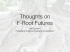### IT – AN ENERGY HOG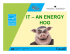### - Lecturer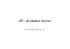### GoAnywhere Director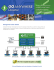### Interactive TV via Internet Protocol (IP TV)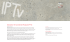### Bob Davis 11/5/2015 Computer Club Presentation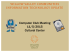### DriveSavers RAID Recovery Services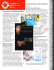### here - Torus Bicycles